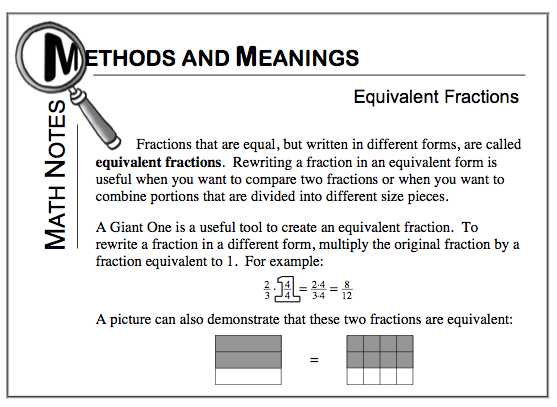Home > CC1MN > Chapter cc21 > Lesson cc21.2.8 > Problem1-137

1-137.

Rewrite each fraction in at least two different ways. Homework Help ✎

1. $\frac { 6 } { 9 }$

1. $\frac { 11 } { 12 }$

1. $\frac { 3 } { 8 }$

1. $\frac { 10 } { 7 }$